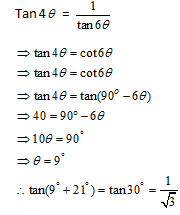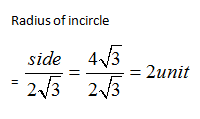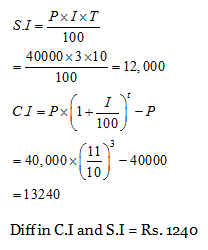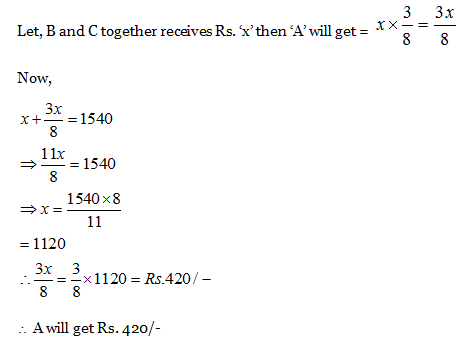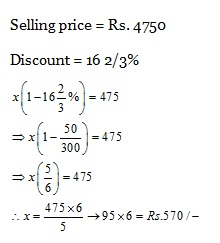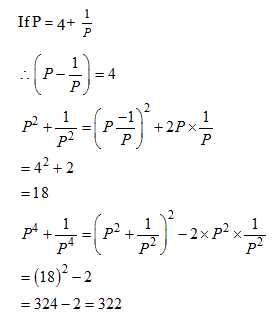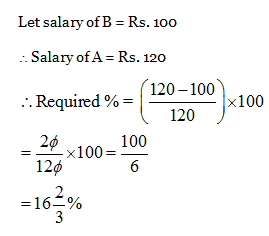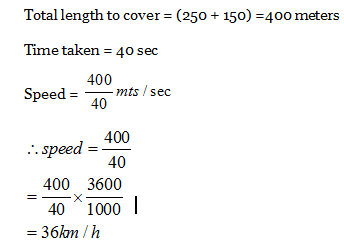# SSC CGL EXAMS 2018 | Quantitative Aptitude Practice Questions (Day-5)

Dear Aspirants, Here we have given the Important SSC Exam 2018 Practice Test Papers. Candidates those who are preparing for SSC 2018 can practice these questions to get more confidence to Crack SSC 2018 Examination.

[WpProQuiz 4100]

1) A circular paddy field of radius 14 meter is divided into three brothers in ratio of 2:1: 1 How much square meter area will the larger party get?

a) 154 sq. meters

b) 308 sq. meters

c) 196 sq.meters

d) 28 sq.meters

2) What is last digit of given expression?

(2193)41 × (625)47 × (218)45

a) 3

b) 5

c) 8

d) 0

3)  A boy can do a work in 3 hours. Three girls can do the same work in 6 hours. In how much time one boy and six girls together can do the same work?

a) 1.5 hours

b) 3 hours

c) 6 hours

d) 2 hours

4)  Which of the following is correct for the statement that if 0 (I.e.  is very small)

a) Sin θ = 1

b) Sin θ = 2

c) Sin θ = 0

d) Sin θ = 1/2

5)  If sum of two numbers are 23, and difference between their square is 207. Find the numbers.

a) 17 and 8

b) 16 and 7

c) 15 and 6

d) 23 and 14

6) What is last digit of given expression?

(24)216 × (313)216 × (47)216

a) 4

b) 1

c) 2

d) 6

7)  The average of 35 numbers is calculated as 20. It is discovered later on that while calculating one number namely 24 was wrongly read as 42 the correct average is:

a) 20.48

b) 19.48

c) 18.48

d) 17.48

8) If tan 4θ. tan 6θ = 1 then the value of tan (θ + 21) is

a) √3

b) 1

c) 1/ √3

d) 0

9) The radius of incircle of an equilateral ∆ABC of side 4√3 unit is x cm. The value of x is

a) 1/3 unit

b) 2 unit

c) √3 unit

d) 3 unit

10) The difference between the simple interest and compound interest (compounded annually) on 40,000 for 3 yrs at 10% per annum

a) 1200

b) 1240

c) 1060

d) 1260

11) A sum of Rs. 1540 has been divided among A, B and C in such a way that A receives 3/8th of what B and C together receives. Then A’s share is-

a) Rs. 420

b) Rs. 540

c) Rs. 380

d) Rs. 1120

12) A Shopkeeper sold an article for Rs.4750/-, after allowing discount of 16 2/3% on the printed price find the printed price.

a) Rs. 540

b) Rs. 570

c) Rs. 590

d) Rs. 585

13)  If (P = 4 + 1/p) then the value of (p4 + 1/p) is

a) 18

b) 318

c) 322

d) 256

14) A’s salary is 20% Higher than B. What percentage B’s salary is lower than that of ‘A’?

a) 16 2/3 %

b) 20%

c) 25%

d) 18%

15) A train of 250 meters length crosses a platform of 150 meters length in 40 sec. What is the speed of train?

a) 40 km/h

b) 36 km/h

c) 72 km/h

d) Cannot be determined

Area of circular field

= πr2

= 22/ 7 * 14 * 14

= 616 sq. meters.

Let, areas are 2x, x, x

Now,

2x + x + x = 616

X = 616/4 = 154 m2

Larger Area = (154 2) = 308 m2

Last digit of (2193)41 = 3

Last digit of (625)47 = 5

Last digit of (218)45 = 8

Last digit of Multiple = ‘0’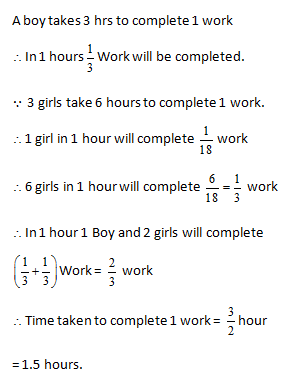For θ = 0

Sin θ = 0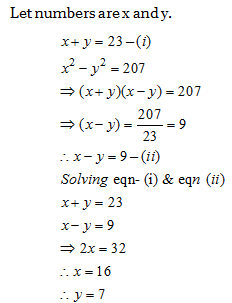Last digit of (24)216 = 6

Last digit of (313)216 = 1

Last digit of (47)216 = 1

Last digit of Multiple = 6  1 1 =6

Total Sum = 35 20 =700

Correct sum should be = 700 – 42 + 24 = 682

Average = 682 / 35 = 19.48